# Lecture 13

## Recursion vs Iteration

MCS 275 Spring 2022
David Dumas

### Lecture 13: Recursion vs Iteration

Course bulletins:

• Anonymous feedback survey to be opened this evening
• Project 1 grades and solutions coming tomorrow
• Project 2 will be posted Monday, due Feb 25

## Paper folding sequence

Last time we discussed pfs(n), a sequence of $2^n-1$ binary digits that encode the directions of ridges in a strip of paper that is folded $n$ times in the same direction and then unfolded.

pfs(n) appears at the beginning of pfs(n+1), so there is also an infinite sequence that is the "limit" of pfs(n) as $n \to \infty$.

## Cool fact

If you use the infinite paper folding sequence as the binary digits of a real number, you get the paper folding constant.

$$\begin{split} PFC &= (0.11011001110010011101100\ldots)_2\\ &= 0.85073618820186\ldots \end{split}$$

This number is irrational. In 2007 it was shown1 that it is also transcendental, i.e. cannot be expressed in terms of square roots, cube roots, or any solutions of polynomials with rational coefficients.

## Stack overflow

Recursive functions are limited by a maximum call stack size.

Python imposes a limit to prevent the memory area used to store the call stack from running out (a stack overflow), which would abruptly stop the interpreter.

## Iterative solutions

Let's write iterative versions of factorial, Fibonacci, and paper folding. (Or as many as time allows.)

## Timing comparison

Let's compare the running time of the iterative and recursive solutions.

## Question

Why is recursive fact() somewhat competitive, but fib() is dreadfully slow?

Decorator decs.count_calls will keep track of number of function calls.

## fact call graph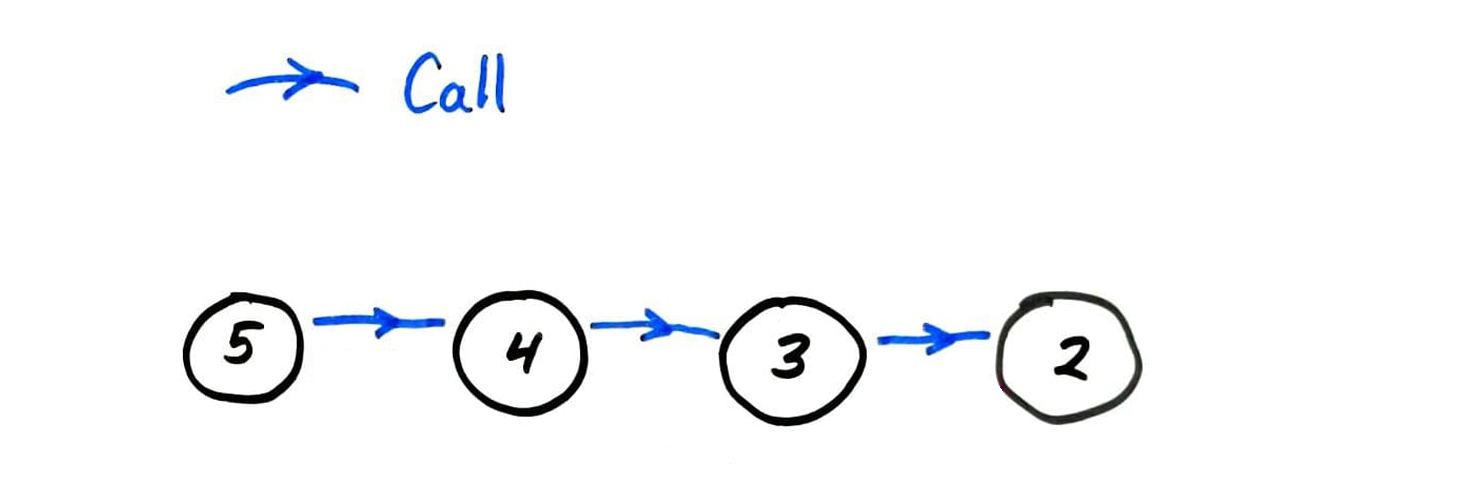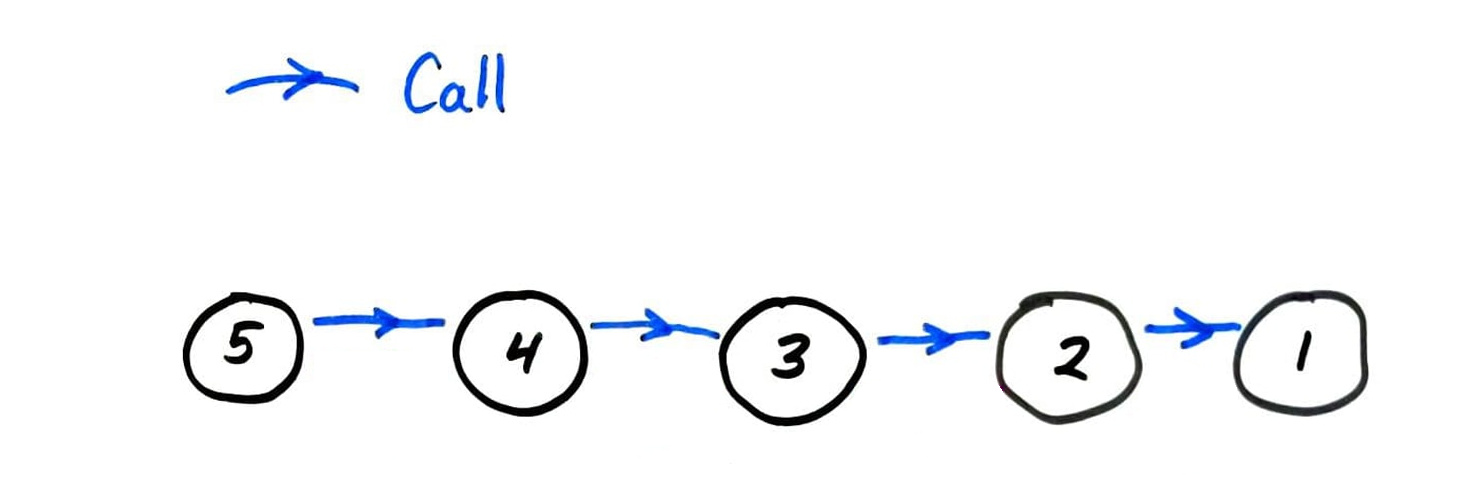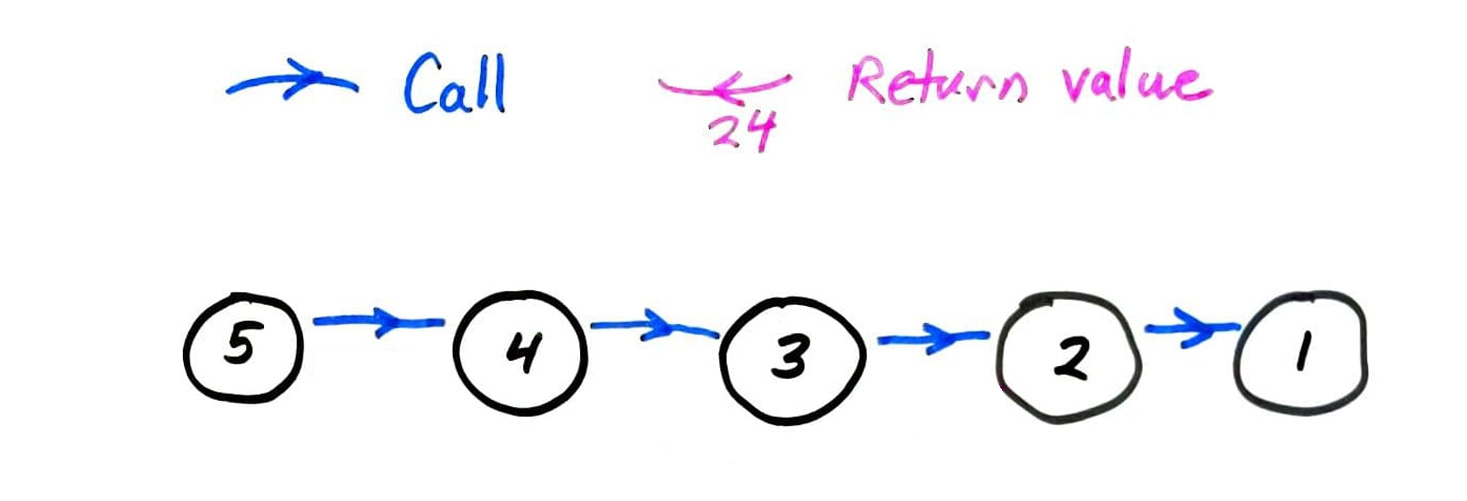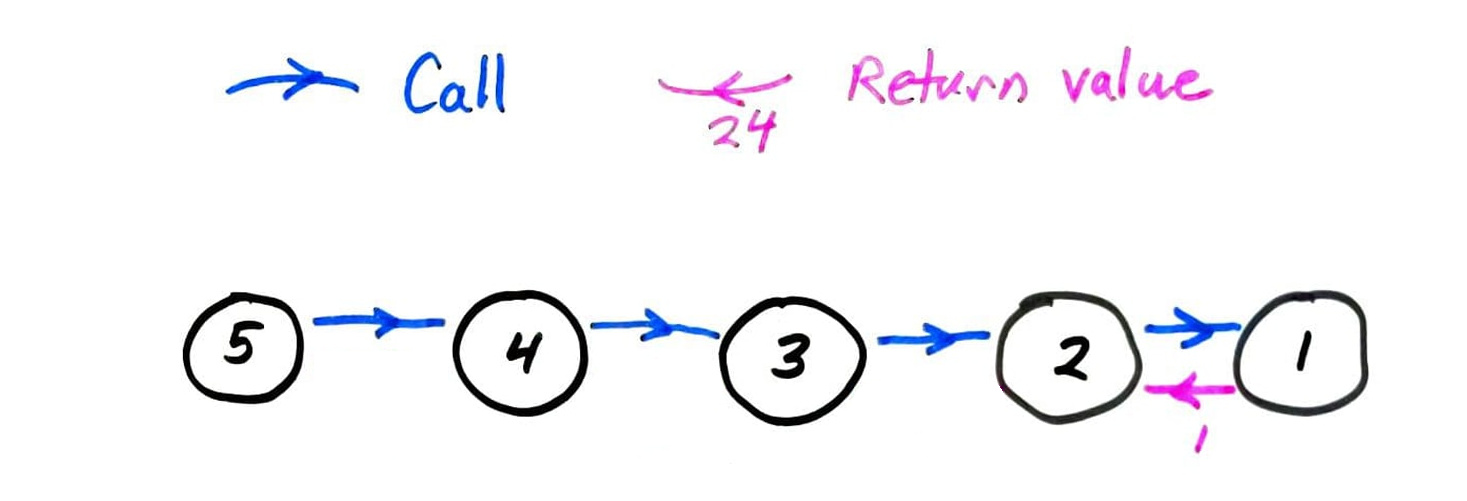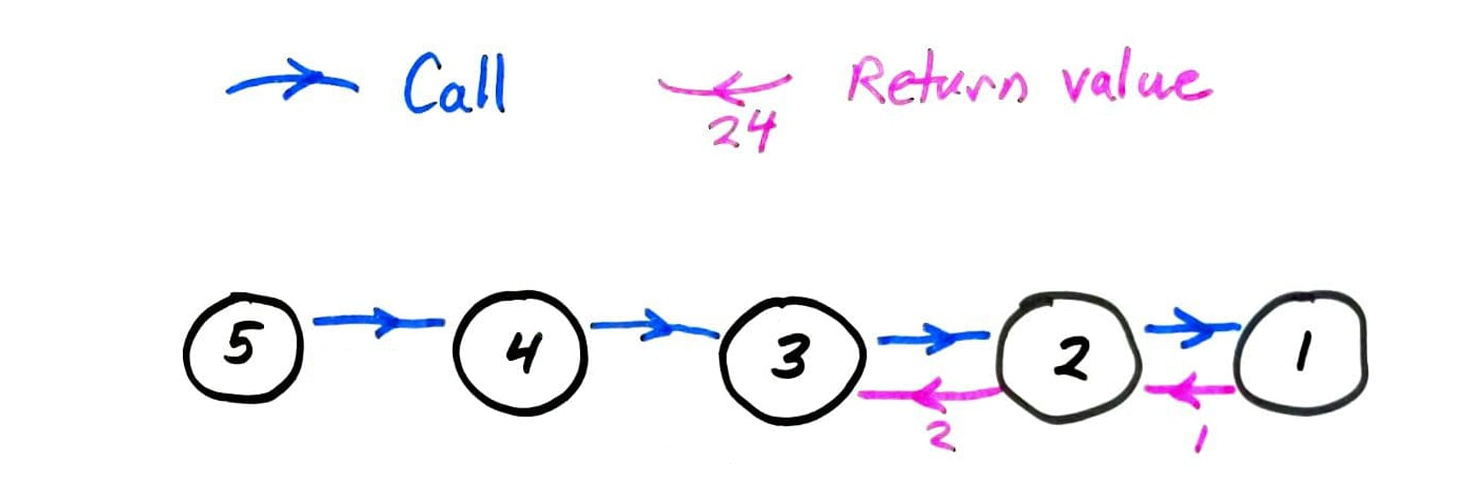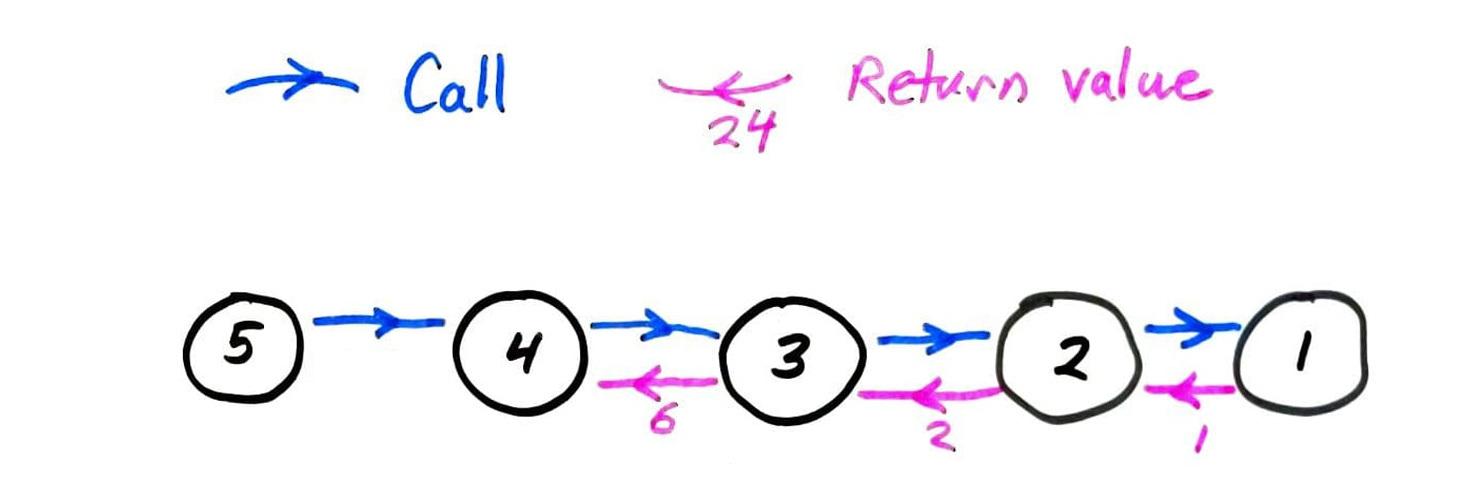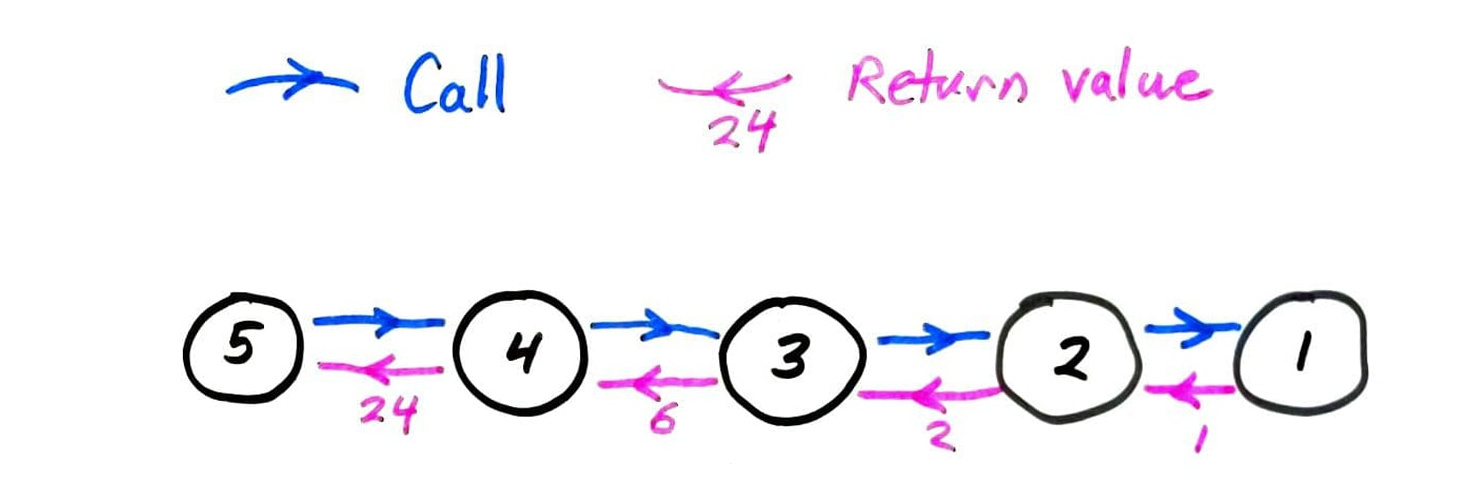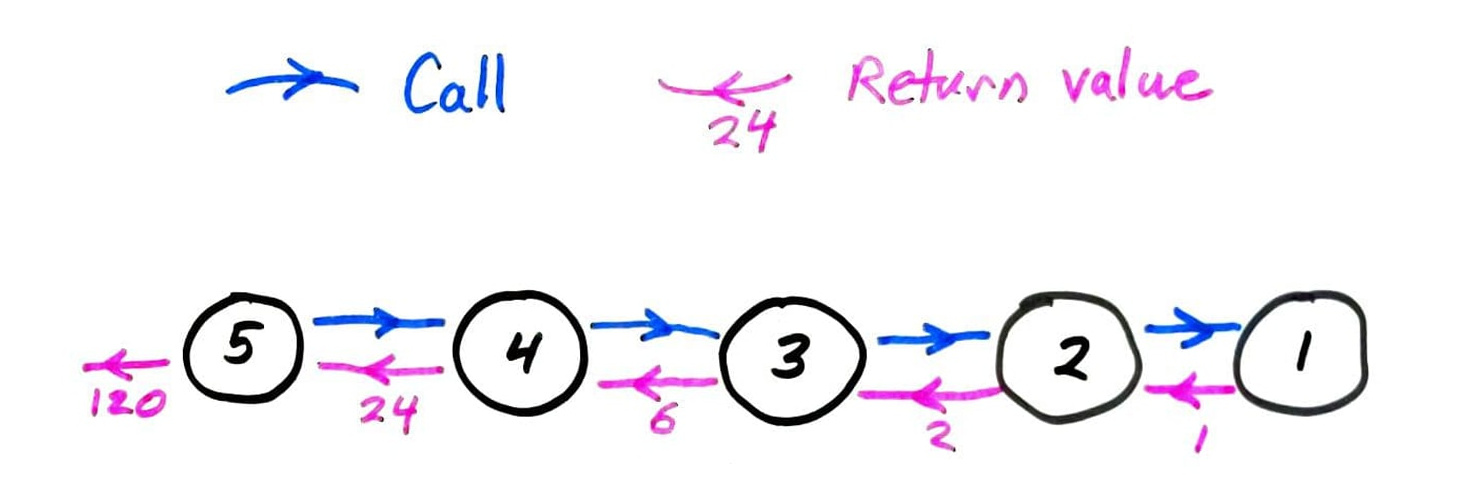## Memoization

fib computes the same terms over and over again.

Instead, let's store all previously computed results, and use the stored ones whenever possible.

This is called memoization. It only works for pure functions, i.e. those which always produce the same return value for any given argument values.

math.sin(...) is pure; random.random() is not.

## Fibonacci timing summary

n=35n=450
recursive1.9s> age of universe
memoized recursive<0.001s0.003s
iterative<0.001s0.001s

Measured on a 4.00Ghz Intel i7-6700K CPU (2015 release date) with Python 3.8.5

## Memoization summary

Recursive functions with multiple self-calls often benefit from memoization.

Memoized version is conceptually similar to an iterative solution.

Memoization does not alleviate recursion depth limits.

Memoization trades running time for memory consumption.

### Revision history

• 2022-02-09 Initial publication